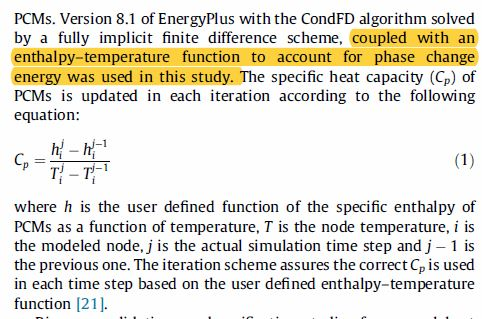Is it possible to use weather data for Parametric simulations?I specifically want to use the values for temperature..

There's this formula described by this study( https://www.researchgate.net/publicat...) where it describes how to calculate the Specific heat capacity (J/kg K) of a phase change material as a function of temperature (specifically, it was referring to 'node temperature', I'm not sure what that means but was it referring to the temperature values from the weather file? or surface temperature perhaps?).

How does one link weather file data or output data to Parametrics? Or did I interpret the equation incorrectly?

Here's a snapshot of the study reffering to this equation:Or maybe it happens automatically? (I think it should, but the author was implying that it doesn't). The values I'm getting from my simulations are quite weird so I'm wondering if this is where I went wrong.

Thanks!

edit retag close merge delete